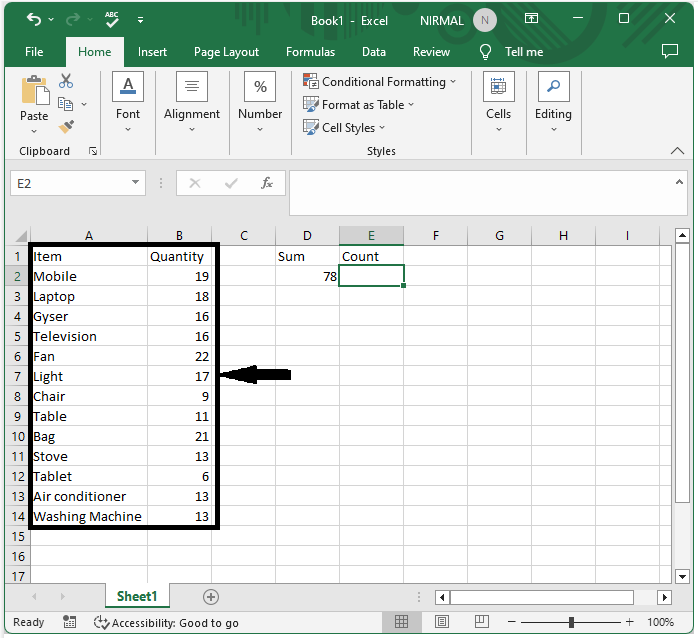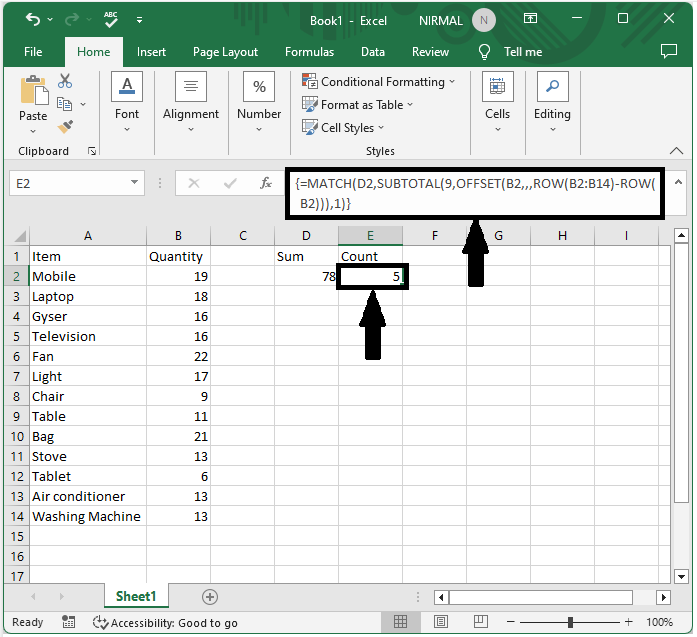# How To Count Rows Until A Certain Sum Value Is Reached In Excel?

Excel is a powerful tool that allows users to perform a wide range of data analysis tasks with ease. One common task is to count the number of rows in a spreadsheet until a certain sum value is reached. This can be useful for various applications, such as calculating the number of sales needed to reach a certain revenue target or determining the number of hours worked until a certain billable amount is reached.

In this tutorial, we will guide you step-by-step on how to count the rows in an Excel spreadsheet until a certain sum value is reached. We will cover different techniques and functions that you can use to achieve this goal, including the SUM function, the COUNT function, and the IF function. Whether you are a beginner or an experienced Excel user, this tutorial will provide you with the knowledge and skills you need to efficiently count rows until a specific sum value is reached in Excel.

## Count Rows Until A Certain Sum Value Is Reached

Here we can get the result directly using a single formula. So let us see a simple process to know how you can count rows until a certain sum value is reached in Excel.

### Step 1

Consider an Excel sheet where you have a list of numbers similar to the below image.Now first click on an empty cell in our case cell S2 and enter the formula as

=MATCH(D2,SUBTOTAL(9,OFFSET(B2,,,ROW(B2:B14)-ROW(B2))),1) and

click Ctrl + Shift + Enter to complete the task.

In the formula, B2:B12 is the range of numbers, and D2 is the address of the required sum.

Empty cell > Formula > CTRL + SHIFT + ENTER## Conclusion

In this tutorial, we used a simple example to demonstrate how you can count rows until a certain sum value is reached in Excel to highlight a particular set of data.

Updated on: 12-Jul-2023

167 Views